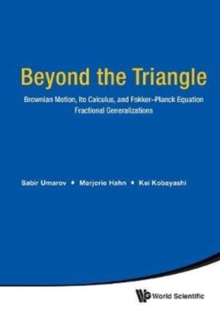Supporting your high street Find out how »
• My Account# Beyond The Triangle: Brownian Motion, Ito Calculus, And Fokker-planck Equation - Fractional Generalizations Hardback

#### Description

The book is devoted to the fundamental relationship between three objects: a stochastic process, stochastic differential equations driven by that process and their associated Fokker-Planck-Kolmogorov equations.

This book discusses wide fractional generalizations of this fundamental triple relationship, where the driving process represents a time-changed stochastic process; the Fokker-Planck-Kolmogorov equation involves time-fractional order derivatives and spatial pseudo-differential operators; and the associated stochastic differential equation describes the stochastic behavior of the solution process.

It contains recent results obtained in this direction.This book is important since the latest developments in the field, including the role of driving processes and their scaling limits, the forms of corresponding stochastic differential equations, and associated FPK equations, are systematically presented.

Examples and important applications to various scientific, engineering, and economics problems make the book attractive for all interested researchers, educators, and graduate students.

#### Information

• Format: Hardback
• Pages: 192 pages
• Publisher: World Scientific Publishing Co Pte Ltd
• Publication Date:
• Category: Differential calculus & equations
• ISBN: 9789813230910

£86.00

£70.19